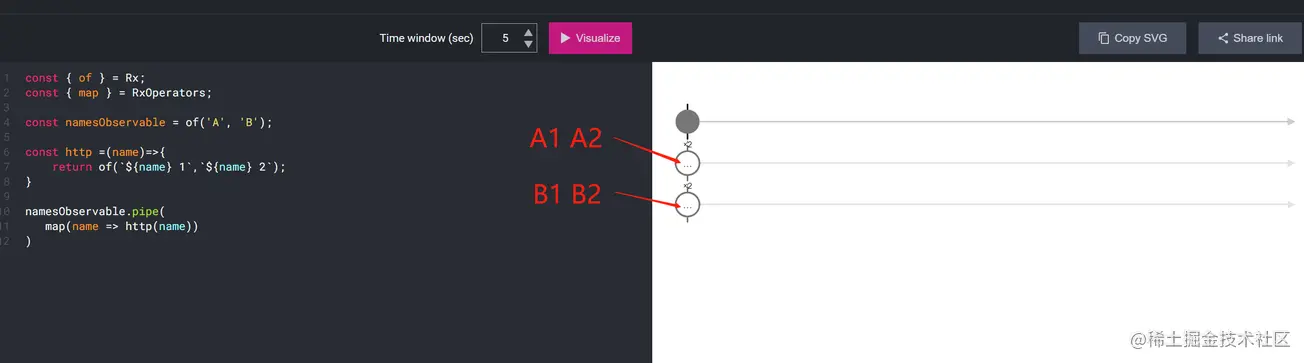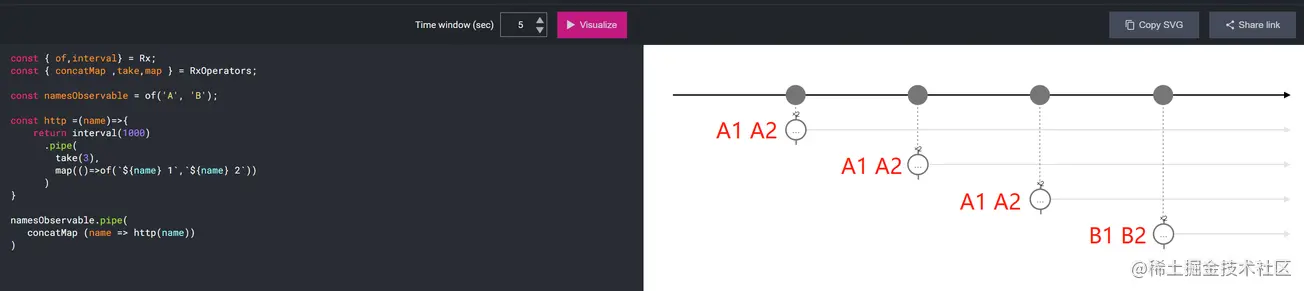# 5 marbles to thoroughly understand the leveling strategy of rxjs: mergemap, switchmap, concatmap and exhaustmap

2022-04-29 20:30:56

RxJS The operator of is really complex to understand , For example, the most commonly used map The operator , In this article, let's rush at them ！！• Original article , For non-commercial reprint, please name the source

map The operation must be familiar to everyone ：

``````const { of } = Rx;
const { map  } = RxOperators;

const namesObservable = of('A', 'B');
namesObservable.pipe(
map(name => `map \${name}`)
)

namesObservable .subscribe(result => console.log(`\${result}`))

// map A
// map B
Copy code ``````

intuitive , because map Mapped is “ value ”, So it's simple enough ~

however , if ,map Mapped is observable Well ？

``````const { of } = Rx;
const { map } = RxOperators;

const namesObservable = of('A', 'B');

const http =(name)=>{
return of(`\${name} 1`,`\${name} 2`);
}

namesObservable.pipe(
map(name => http(name))
)

namesObservable.subscribe(result => console.log(`\${result}`))

//  You'll get two  observable  object
// ****observable{ .. }
// observable{ .. }
Copy code ``````

We are rxviz.com/ In the picture of marbles , Can be seen clearly ： It is still observablealso observable From the original 1 individual , Turned into 2 individual （ The circle is observable）, The data is still inside and has not been parsed by the subscription .

although , We can use Brutal Methods , The subscription `.subscribe` Call the subscription again `.subscribe` , Then the value can be obtained ：

``````const { of } = Rx;
const { map } = RxOperators;

const namesObservable = of('A', 'B');

const http =(name)=>{
return of(`\${name} 1`,`\${name} 2`);
}

namesObservable.pipe(
map(name => http(name))
)

namesObservable .subscribe(resultObservable => {
resultObservable.subscribe(result => console.log(`\${result}`) )
})

// A1
// A2
// B1
// B2
Copy code ``````

however , This kind of package writing is doomed to be inelegant , therefore , To solve this difference ,RxJS Introduced —— Flattening（ flat ） Strategy ！！

We can use flatMap The operator , The same analytical value can be obtained ~

flatMap In fact, we are well-known mergeMap The operator ;

The code is as follows ：

``````const { of } = Rx;
const { mergeMap} = RxOperators;

const namesObservable = of('A', 'B');

const http =(name)=>{
return of(`\${name} 1`,`\${name} 2`);
}

namesObservable.pipe(
mergeMap(name => http(name))
)

namesObservable.subscribe(result => console.log(`\${result}`))
// A1
// A2
// B1
// B2
Copy code ``````

Further more , Follow this kind of flattening strategy , except mergeMap,RxJS And the introduction of switchMap、concatMap and exhaustMap, They can provide leveling strategies in different directions .

Let's use rxviz.com/ Marbles map , You can see their differences at a glance ：

Set a timer , Every second sends a observable, A total of 3 Time , Let's take a look at the respective values ;

• mergeMap
``````const { of,interval} = Rx;
const { mergeMap,take,map } = RxOperators;

const namesObservable = of('A', 'B');

const http =(name)=>{
return interval(1000)
.pipe(
take(3),
map(()=>of(`\${name} 1`,`\${name} 2`))
)
}

namesObservable.pipe(
mergeMap(name => http(name))
)
Copy code ```````mergeMap` Internal subscriptions for multiple activities will be maintained at the same time ;

• switchMap
``````const { of,interval} = Rx;
const { switchMap,take,map } = RxOperators;

const namesObservable = of('A', 'B');

const http =(name)=>{
return interval(1000)
.pipe(
take(3),
map(()=>of(`\${name} 1`,`\${name} 2`))
)
}

namesObservable.pipe(
switchMap(name => http(name))
)
Copy code ```````switchMap`, Every time it's sent out , Will cancel the previous internal observable The subscription , Then subscribe to a new observable;

• concatMap
``````const { of,interval} = Rx;
const { concatMap ,take,map } = RxOperators;

const namesObservable = of('A', 'B');

const http =(name)=>{
return interval(1000)
.pipe(
take(3),
map(()=>of(`\${name} 1`,`\${name} 2`))
)
}

namesObservable.pipe(
concatMap (name => http(name))
)
Copy code ```````concatMap` Will be in the previous interior observable After completion , Will subscribe to the next ;

• exhaustMap
``````const { of,interval} = Rx;
const { exhaustMap ,take,map } = RxOperators;

const namesObservable = of('A', 'B');

const http =(name)=>{
return interval(1000)
.pipe(
take(3),
map(()=>of(`\${name} 1`,`\${name} 2`))
)
}

namesObservable.pipe(
exhaustMap (name => http(name))
)
Copy code ```````exhaustMap` Map to internal observable, Ignore other values until this observable complete ;